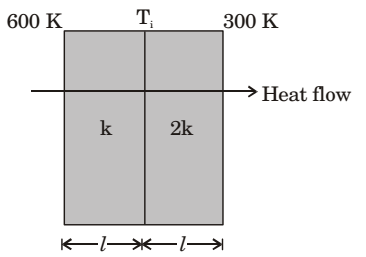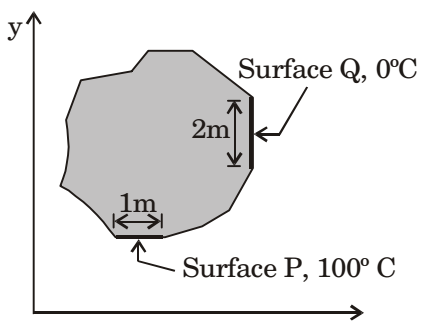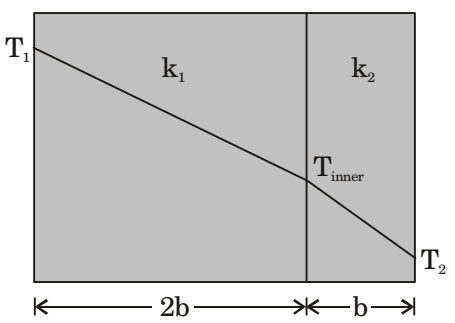## Heat Transfer Miscellaneous

#### Heat-Transfer

1. In pool boiling the highest HTC occurs in

1. Nucleate boiling zone

##### Correct Option: B

Nucleate boiling zone

1. Heat transfer through a composite wall is shown in figure. Both the sections of the wall have equal thickness (/). The conductivity of one section is k and that of the other is 2k. The left face of the wall is at 600 K and the right face is at 300 K.The interface temperature Ti (in K) of the composite wall is __________

1. R = R1 + R2

 = L + L = L + L = 3L KA KA KA 2KA 2KA

 Q = TL - TR TR

 = 300 = 200 × KA 3L L 2KA

At interface,
 Q = TL - Ti Ti

 = 600 - Ti = (600 - Ti) KA L L KA

 200 KA = (600 - Ti) KA L L

200 = 600 – Ti
Ti = 400 K

##### Correct Option: A

R = R1 + R2

 = L + L = L + L = 3L KA KA KA 2KA 2KA

 Q = TL - TR TR

 = 300 = 200 × KA 3L L 2KA

At interface,
 Q = TL - Ti Ti

 = 600 - Ti = (600 - Ti) KA L L KA

 200 KA = (600 - Ti) KA L L

200 = 600 – Ti
Ti = 400 K

1. Steady two-dimensional heat conduction takes place in the body shown in the figure below. The normal temperature gradients over surface P and Q can be considered to be uniform. The temperature gradient δT/δt at surface Q is equal to 10 K/m. Surfaces P and Q are maintained at constant temperatures as shown in the figure, while the remaining part of the boundary is insulated. The body has a constant thermal conductivity of 0.1 W/mK.
 The values of δT and δT at surface P are δy δx1. Direction of heat flow is always normal to surface of constant temperature
So for surface P1, dT/dx = 0
From energy conservation,
Heat rate at P = Heat rate at Q

 0.1 × 1dT= 0.1 × 2 ×dTdy P dx Q

 ⇒ dT = 20 K/m dy

##### Correct Option: D

Direction of heat flow is always normal to surface of constant temperature
So for surface P1, dT/dx = 0
From energy conservation,
Heat rate at P = Heat rate at Q

 0.1 × 1dT= 0.1 × 2 ×dTdy P dx Q

 ⇒ dT = 20 K/m dy

1. Heat is being transferred conductivity from a cylindrical nuclear reactor fuel rod of 50 mm diameter to water at 75°C, under steady state condition, the rate of heat generation within the fuel element is 106 W/m3 and the convective heat transfer coefficient is 1 kW/m2K, the outer surface temperature of the fuel element would be

1. By applying energy balance, we get
Rate of heat generation = Rate of convective heat transfer
qG × Volume of fuel rod = h0 A (Ts – T0)

 qQ × π d2 × 1 = h0 × πdl(Ts – T0) 4

 d.qQ = h0(Ts – T0) 4

 0.05 × 1 × 103 (Ts – 75) 4

Ts – 75 = 12.5
Ts = 87.5°C or 360.5K

##### Correct Option: C

By applying energy balance, we get
Rate of heat generation = Rate of convective heat transfer
qG × Volume of fuel rod = h0 A (Ts – T0)

 qQ × π d2 × 1 = h0 × πdl(Ts – T0) 4

 d.qQ = h0(Ts – T0) 4

 0.05 × 1 × 103 (Ts – 75) 4

Ts – 75 = 12.5
Ts = 87.5°C or 360.5K

1. In a composite slab, the temperature at the interface (Tinter) between two materials is equal to the average of the temperature at the two ends. Assuming steady one dimensional heat conduction, which of the following statements is true about the respective thermal conductivity?1.  Q = -k1A (Tinter - T1) = -k2A(T2 - Tinter) 2b b

 Tinter = T1 + T2 2

∴ k1 = 2k2

##### Correct Option: D

 Q = -k1A (Tinter - T1) = -k2A(T2 - Tinter) 2b b

 Tinter = T1 + T2 2

∴ k1 = 2k2